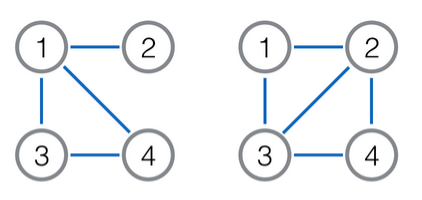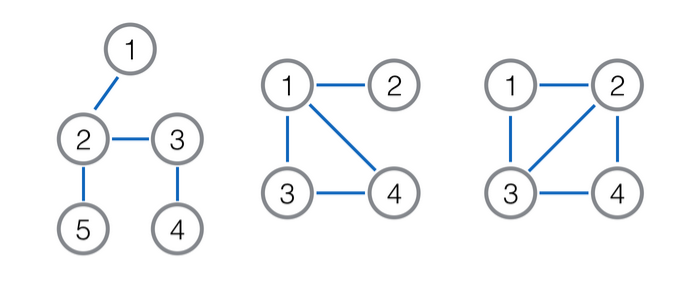# #373. 【ZJOI2018】线图

• 它的点集大小为 $E$，每个点唯一对应着原图的一条边。
• 两个点之间有边当且仅当这两个点对应的边在原图上有公共点(注意不会有自环)。### 样例一

#### input

5 3
1 2
2 3
2 5
3 4



#### output

5



#### explanation### 数据范围与约定

$2\le n\le 5000$。

1 $=2$
2 $=3$
3 $=4$
4 $=5$
5 $=5$
6 $=6$
7 $=7$
8 $=8$
9 $=9$
10 $=9$